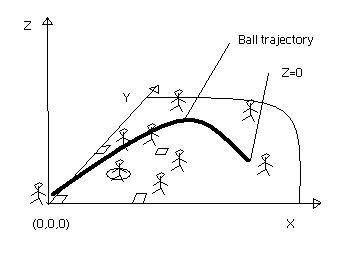Problem E

Play Ball

Source code: playball.*While on a trip to the US, you decide to stop by Atlanta and get to the baseball stadium where the Braves are playing a decisive baseball game against the NY Mets.

According to the players' position on the field, they may catch the balls that are hit by the batters, if they can get there on time before the ball touches the ground. The ball's position follows the following simple mathematical equations:For the ball:   z = at2+bt + c    -  z is the height of the ball above the ground.   x = dt+e    -  x is the position along the x-axis.   y = ft+g    -  y is the position along the y-axis.    -  a,b,..,g are constants given for each ball hit.    -  t is the time elapsed since the ball was hit.

Each player has an individual starting position (xo, yo) he always returns to before a new ball is hit. The players, each starting to run as soon as the ball is hit towards the correct position where the ball will eventually touch the ground, all theoretically have a chance to catch the ball. So you must verify all of them since the distance they can run is dependant on their individual speed. Well, after all, we assume that these players always run as fast as possible given the good paychecks they are given, so the variation of their position is always the same throughout the match, e.g. they run at the same speed.

Important notes:

• A ball that ends up touching the ground elsewhere than in both positive side of the ground axis (x and y) is said to be "foul" and can not be caught. These cases are trivial but must be taken care of.
• The time the ball takes to travel (t) is the smallest integer that satisfies the equation of the height of the ball, i.e. t is the first positive integer for which z becomes zero or negative. This integer is the time when the ball is considered to hit the ground.
• A ball "not caught" before it touches the ground is said to be "safe".
• The maximum number of players is less than 20 players, but could be any number below that limit.
The Input:

The format of the input is the following (where all parameters are integer values):

PLAYERS=#                             // number of players/lines to read
x y v                                           // x,y - startup position of the player; v - the speed of the player
...                                                // for each player
BALLS=#                                  // Number of balls that are hit
a b c d e f g                                // Parameters according to the ball equations
...                                                // for each ball

A sample input:

```PLAYERS=9
0 0 3
16 16 2
30 0 4
36 24 4
0 30 3
24 36 5
24 75 7
75 24 6
60 60 7
BALLS=12
-1 3 1 21 3 14 5
-1 1 1 11 3 2 5
-3 4 0 8 5 11 2
-1 2 1 -4 1 6 7
-1 1 0 9 3 14 5
-2 4 1 32 3 12 5
-1 1 1 11 1 19 5
-4 5 0 18 5 20 5
-1 2 1 24 5 6 7
-1 1 0 9 3 4 1
-2 2 1 2 5 22 5
-1 6 1 10 5 13 5
```

The output:

The output should consist of one line per ball following the format:
"Ball i was ??? at (xkor,ykor)", where i is the ball number, ??? is one of caught, safe, or foul, and (xkor,ykor) is the coordinates where the ball hit the ground. Look at the example below!

The corresponding output to the sample input above:

```Ball 1 was caught at (87,61)
Ball 2 was safe at (25,9)
Ball 3 was safe at (21,24)
Ball 4 was foul at (-11,25)
Ball 5 was safe at (12,19)
Ball 6 was safe at (99,41)
Ball 7 was caught at (23,43)
Ball 8 was safe at (41,45)
Ball 9 was caught at (77,25)
Ball 10 was safe at (12,5)
Ball 11 was safe at (9,49)
Ball 12 was caught at (75,96)

```